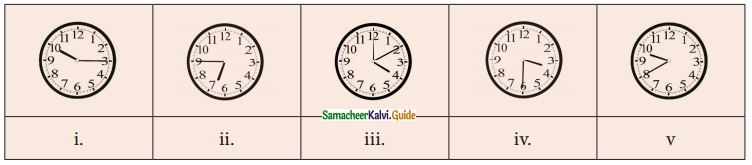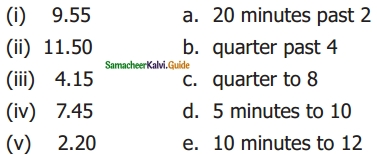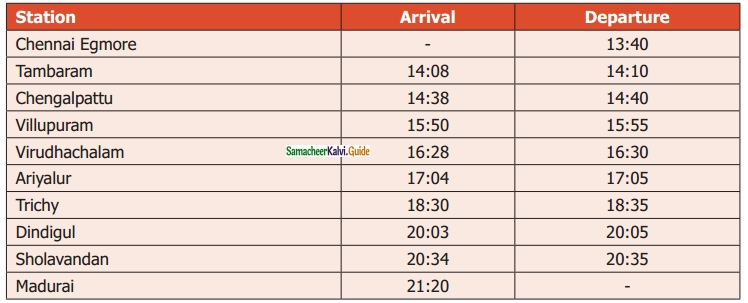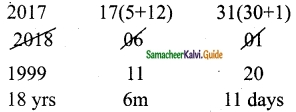Students can download Maths Chapter 2 Measurements Ex 2.2 Questions and Answers, Notes, Samacheer Kalvi 6th Maths Guide Pdf helps you to revise the complete Tamilnadu State Board New Syllabus, helps students complete homework assignments and to score high marks in board exams.

## Tamilnadu Samacheer Kalvi 6th Maths Solutions Term 2 Chapter 2 Measurements Ex 2.2

Question 1.
Say the time in two ways:Solution:
(i) 10 : 15 hours; quarter past 10; 45 minutes to 11
(ii) 6 : 45 hours; quarter to 7; 45 minutes past 6
(iii) 4 : 10 hours; 10 minutes past 4; 50 minutes to 5
(iv) 3 : 30 hours; half-past 3; 30 minutes to 4
(v) 9 : 40 hours; 20 minutes to 10; 40 minutes past 9.Question 2.
Match the following:Solution:
(i) d
(ii) e
(iii) b
(iv) c
(v) aQuestion 3.
Convert the following:
(i) 20 minutes into seconds
(ii) 5 hours 35 minutes 40 seconds into seconds
(iii) 3 ½ hours into minutes
(iv) 580 minutes into hours
(v) 25200 seconds into hours
Solution:
(i) 20 minutes into seconds:
1 min = 60 seconds
20 min = 20 × 60 seconds
= 1200 seconds

(ii) 5 hours 35 min 40 seconds into seconds
Solution:
1 hour = 60 min
1 min = 60 seconds
1 hour = 3600 seconds
5 hours = 5 × 3600 seconds
= 18000 seconds
35 min = 35 × 60 seconds
= 2100 seconds
5 hours 35 minutes 40 seconds
= (18000 + 2100 + 40) seconds
= 20140 seconds(iii) 3 ½ hours into minutes
Solution:
1 hour = 60 minutes
3 ½ hours = 3 hours + 30 min
= (3 × 60 + 30) min
= (180 + 30)min
= 210 min

(iv) 580 minutes into hours
Solution:
1 hour = 60 min
580 min
= $$\frac{580}{60}$$ hours
= $$\frac{290}{30}$$ hours
= $$\frac{29}{3}$$
= 9 $$\frac{2}{3}$$
= 9 hours 40 min

(v) 25200 seconds into hours:
Solution:
25200 seconds = $$\frac{25200}{3600}$$
= $$\frac{126}{18}$$ hours
= $$\frac{63}{9}$$ hours
= 7 hoursQuestion 4.
The duration of electricity consumed by the farmer for his pump set on Monday and Tuesday was 7 hours 20 minutes 35 seconds and 3 hours 44 minutes 50 seconds respectively. Find the total duration of consumption of electricity.
Solution:
The total duration of electricity consumed on both days
= 7 hours 20 min 35 sec + 3 hours 44 min 50 sec
= (7 + 3) hours (20 + 44) min (35 + 50) sec
= 10 hours 64 min 85 seconds
= 11 hours 5 min 25 seconds

Question 5.
Subtract 10 hours 20 min 35 seconds from 12 hours 18 min 40 seconds.
Solution:
12 hours 18 min 40 seconds
= (12 × 3600) + (18 × 60) + 40 seconds
= 43200 + 1080 + 40 seconds
= 44320 seconds
10 hours 20 min 35 seconds
= (10 × 3600) + (20 × 60) + 35 seconds
= 36000 + 1200 + 35 seconds
= 37235 seconds
Difference:
44320 – 37235
= 7085
7085 seconds = (1 × 3600) + 3480 + 5 seconds
= 1 hour 58 minutes 5 secondsQuestion 6.
Change the following into 12 hour format
(i) 02:00 hours
(ii) 08:45 hours
(iii) 21:10 hours
(iv) 11:20 hours
(v) 00:00 hours
Solution:
(i) 2 am
(ii) 08:45 am
(iii) 9:10 pm
(iv) 11:20 am
(v) 12 midrid

Question 7.
Change the following into 24-hour format.
(i) 3.15 am
( ii) 12.35 pm
(iii) 12.00 noon
(iv) 12.00 mid night
Solution:
(i) 03.15 hours
(ii) 12.35 hours
(iii) 12.00 hours
(iv) 24.00 hoursQuestion 8.
Calculate the duration of time
(i) from 5.30 am to 12.40 pm
(ii) from 1.30 pm to 10.25 pm
(iii) from 20.00 hours to 4.00 hours
(iv) from 17.00 hours to 5.15 hours
Solution:
(i) from 5.30 a.m. to 12 .40 p.m.
Duration of time from 5.30 a.m. to noon = 12 : 00 – 5 : 30 = 6 : 30 i.e 6 hours 30 minutes
From noon to 12.40 p.m the duration = 00 hours 40 minutes
Total duration = 6 hours 30 minutes + 00 hours 40 minutes
= 6 hours 70 minutes
= 6 hours + (60 + 10) minutes
= 6 hours + 1 hr 10 minutes
= 7 hours 10 minutes
∴ Duration of time from 5.30 am to 12.40 pm = 7 hours 10 minutes

(ii) From 1.30 pm to 10.25 pm
= (1.30 pm to 10.00 pm) + 25 min
= 8 hrs 30 min + 25 min
= 8 hrs 55 min

(iii) From 20.00 hours to 4.00 hours
= (20.00 hrs to 24.00 hrs) + (24.00 hrs to 4.00 hrs)
= 4 hrs + 4 hrs
= 8 hours

(iv) From 17.00 hrs to 5.15 hours
= (17.00 hrs to 05.00 hrs) + 15 min
= 12 hours + 15 min
= 12 hours 1.5 minQuestion 9.
The departure and arrival timing of the Vaigai Superfast Express (No. 12635) from Chennai Egmore to Madurai Junction are given. Read the details and answer the following.(i) At what time does the Vaigai Express start from Chennai and arrive at Madurai?
(ii) How many halts are there between Chennai and Madurai?
(iii) How long does the train halt at the Villupuram Junction?
(iv) At what time does the train come to Sholavandan?
(v) Find the journey time from Chennai Egmore to Madurai?
Solution:
(i) 13.40 hours – 21.20 hours
(ii) 8 halts
(iii) 5 minutes
(iv) 20.34 hours
(v) 7 hours 40 minutesQuestion 10.
Manickam joined a chess class on 20.02.2017 and due to an exam, he left practice after 20 days. Again he continued to practice from 10.07.2017 to 31.03.2018. Calculate how many days did he practice?
Solution:
From the date of joining = 20 days From 10.07.2017 to 31.03.2018
July – 22
Aug – 31
Sep – 30
Oct – 31
Nov – 30
Dec – 31
Jan – 31
Feb – 28
Mar – 31
Total – 265
Total no of practice days = 265 + 20 = 285 days

Question 11.
A clock gains 3 minutes every hour. If the clock is set correctly at 5 am, find the time shown by the clock at 7 p.m?
Solution:
Time gained for 1 hour = 3 min
Time duration from 5 am to 7 pm = 14 hours
Time gained for 14 hours = 1 4 × 3 minutes
= 42 minutes
So, at 7 pm, the clock shows 7 hrs 42 minutesQuestion 12.
Find the number of days between Republic day and Kalvi Valarchi Day in 2020.
Solution:
In 2020 Republic Day will be celebrated on 26th January and Kalvi Valarchi Day will be celebrated on 15th July.
Number of days between 26.01.2020 and 15.07.2020
January – 6 Days (from 26.01.2020)
February – 29 Days (2020 is a leap year)
March – 31 Days
April – 30 Days
May – 31 Days
June – 30 Days
July – 15 Days (upto 15.07.2020)
Total – 172 Days.
∴ Total number of days = 172

Question 13.
If the 11th of Jan 2018 is Thursday, what is the day on 20th July of the same year?
Solution:
Jan – 21
Feb – 28
Mar – 31
April – 30
May – 31
June – 30
July – 19
Total – 190 days
190 days = 27 weeks + 1 day
The required day is the first day after Thursday.
Therefore 20th July 2018 is Friday.Question 14.
(i) Convert 480 days into years.
(ii) Convert 38 months into years
Solution:
(i) 480 days = $$\frac{480}{365}$$
= 1 year 115 days
= 1 year 3 months 25 days

(ii) 38 months = $$\frac{38}{12}$$
= 3 years 2 months

Question 15.
Calculate your age as on 01.06.2018
Solution:
My date of birth 20.11.1999
Convert in the format yyyy/mm/ddMy age is 18 yrs 6 months 11 daysObjective Type Questions

Question 16.
2 days = _____ hours.
(a) 38
(b) 48
(c) 28
(d) 40
Solution:
(b) 48

Question 17.
3 weeks = ……… days
(i) 21
(ii) 7
(iii) 14
(iv) 28
Solution:
(i) 21Question 18.
The number of ordinary years between two consecutive leap years is _____.
(a) 4 years
(b) 2 years
(c) 1 year
(d) 3 years
Solution:
(d) 3 years

Question 19.
What time will it be 5 hours after 22:35 hours?
(i) 2:30 hours
(ii) 3:35 hours
(iii) 4:35 hours
(iv) 5:35 hours
Solution:
(ii) 3:35 hoursQuestion 20.
2$$\frac { 1 }{ 2 }$$ years is equal to ______ months.
(a) 25
(b) 30
(c) 24
(d) 5
Solution:
(b) 30